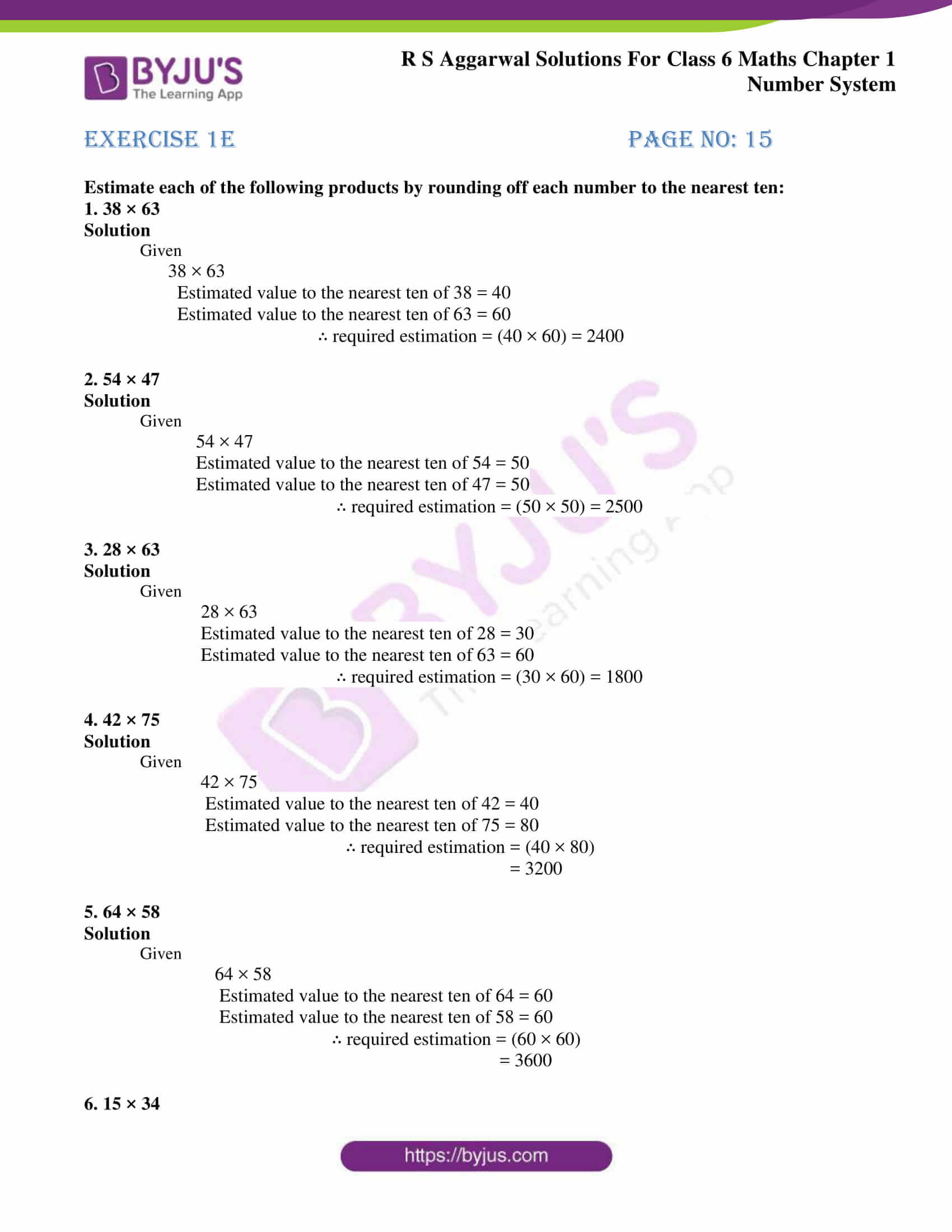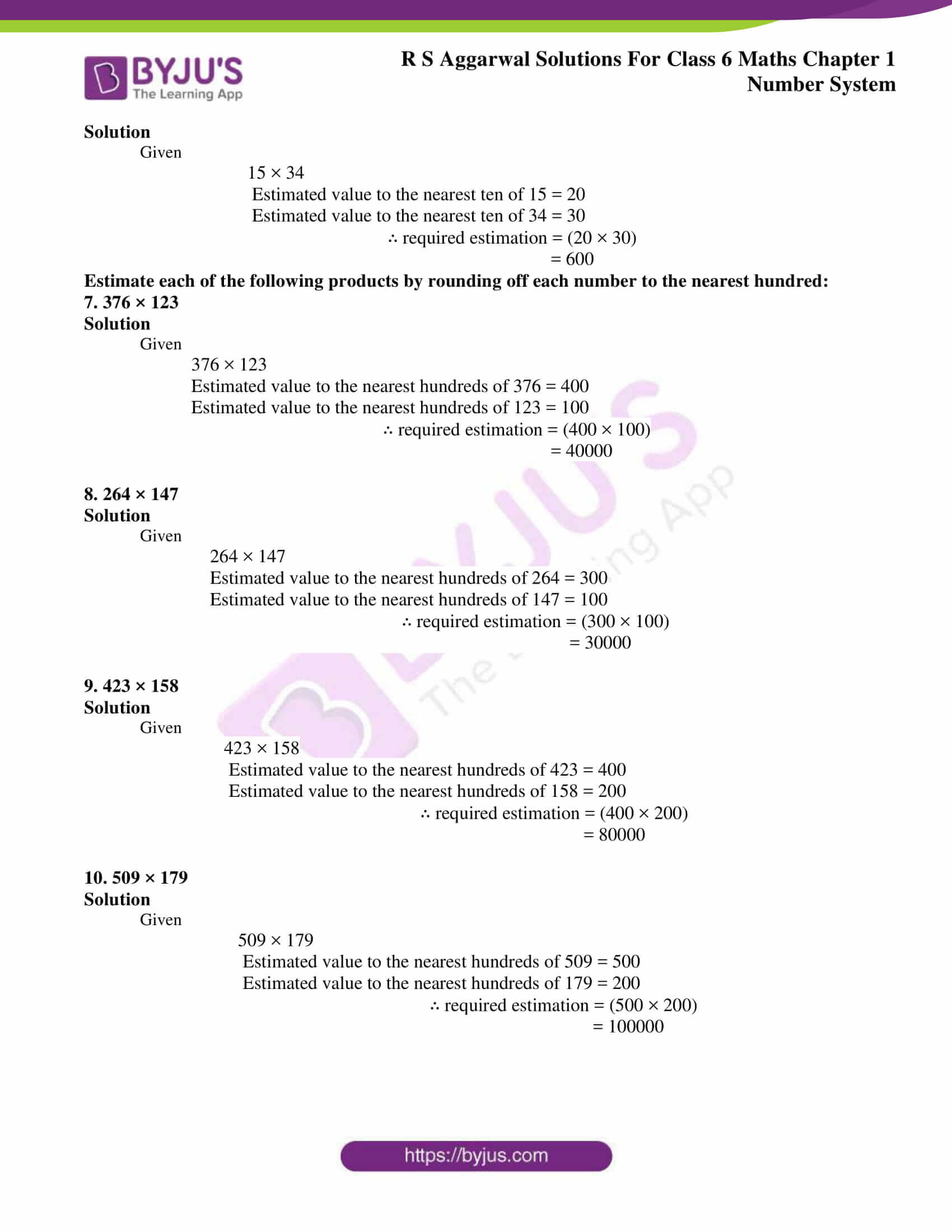# RS Aggarwal Solutions for Class 6 Chapter 1 Number System Exercise 1E

PDF Format of RS Aggarwal Solutions for Class 6 Chapter 1 Number System Exercise 1E are given here. RS Aggarwal Solutions help the students to understand the chapter thoroughly and perform better in the exam. Students who aim to understand the subject with ease are advised to practice problems using the RS Aggarwal Solutions for Class 6

Mathematics experts at BYJU’S have solved questions of RS Aggarwal textbook Exercise 1E for students which are available in PDF. Exercise 1E is based on the topic of estimating products. Those who want to solve the problems of Exercise 1E are advised to go through RS Aggarwal Solutions.

## Download PDF of RS Aggarwal Solutions for Class 6 Chapter 1 Number System Exercise 1E### Access answers to Maths RS Aggarwal Solutions for Class 6 Chapter 1 Number System Exercise 1E

Estimate each of the following products by rounding off each number to the nearest ten:

1. 38 × 63

Solution

Given

38 × 63

Estimated value to the nearest ten of 38 = 40

Estimated value to the nearest ten of 63 = 60

∴ required estimation = (40 × 60) = 2400

2. 54 × 47

Solution

Given

54 × 47

Estimated value to the nearest ten of 54 = 50

Estimated value to the nearest ten of 47 = 50

∴ required estimation = (50 × 50) = 2500

3. 28 × 63

Solution

Given

28 × 63

Estimated value to the nearest ten of 28 = 30

Estimated value to the nearest ten of 63 = 60

∴ required estimation = (30 × 60) = 1800

4. 42 × 75

Solution

Given

42 × 75

Estimated value to the nearest ten of 42 = 40

Estimated value to the nearest ten of 75 = 80

∴ required estimation = (40 × 80)

= 3200

5. 64 × 58

Solution

Given

64 × 58

Estimated value to the nearest ten of 64 = 60

Estimated value to the nearest ten of 58 = 60

∴ required estimation = (60 × 60)

= 3600

6. 15 × 34

Solution

Given

15 × 34

Estimated value to the nearest ten of 15 = 20

Estimated value to the nearest ten of 34 = 30

∴ required estimation = (20 × 30)

= 600

Estimate each of the following products by rounding off each number to the nearest hundred:

7. 376 × 123

Solution

Given

376 × 123

Estimated value to the nearest hundreds of 376 = 400

Estimated value to the nearest hundreds of 123 = 100

∴ required estimation = (400 × 100)

= 40000

8. 264 × 147

Solution

Given

264 × 147

Estimated value to the nearest hundreds of 264 = 300

Estimated value to the nearest hundreds of 147 = 100

∴ required estimation = (300 × 100)

= 30000

9. 423 × 158

Solution

Given

423 × 158

Estimated value to the nearest hundreds of 423 = 400

Estimated value to the nearest hundreds of 158 = 200

∴ required estimation = (400 × 200)

= 80000

10. 509 × 179

Solution

Given

509 × 179

Estimated value to the nearest hundreds of 509 = 500

Estimated value to the nearest hundreds of 179 = 200

∴ required estimation = (500 × 200)

= 100000

## RS Aggarwal Solutions for Class 6 Maths Chapter 1 Number System Exercise 1E

Exercise 1E has the questions based on the concept of Estimating the products. Those who wish to understand the topics clearly are advised to practice RS Aggarwal textbook of Class 6 Chapter 1 Number System Exercise 1E which helps them to score high marks in the examinations. Topics which are included in other exercises are:

• International system of numeration
• Comparison of numbers
• Word problems on number operations
• Estimation
• Estimating the quotients
• Roman numerals

The concepts which are covered in this chapter are explained in simple language to help the students while studying. The solutions PDF help students improve knowledge about concepts and perform better in the exam.